Updated date:

# The Standard Model of Particle Physics: What Is Our Universe Made of?

Author:

Sam Brind holds a master's in physics with theoretical physics (MPhys) from the University of Manchester.

Everything is made up of atoms. This is what you were hopefully taught at school and it was the historical starting point for particle physics. The atom was decomposed and its structure studied in the early 20th century. Beginning to understand the atom was a significant achievement but in the realm of modern physics the atom is too large (about 1x10-10 metres wide) to be described as particle physics.

The standard model is our current theory that best describes particle physics, having been developed throughout the latter half of the 20th century. It is a well established theory, having stood up to significant testing. Indeed it has predicted many particles, to a good precision, before their experimental discovery. The latest confirmation being the discovery of the Higgs boson at the large hadron collider (LHC). The standard model describes the types of particles that make up matter and the possible interactions between these particles.

## Types of Particle

All particles belong to one of two categories: fermions or bosons. The classification of which category a particle belongs to is based on the particle's spin. Spin is an important quantum mechanical property that is intrinsic to the particle and nothing to do with the particle actually physically spinning.

A fermion is any particle that has a half-integer spin (1/2, 3/2, 5/2, etc.). The consequence of this, called the Pauli exclusion principle, is that fermions can't occupy the same quantum state; a quantum state simply being all the values of the particle's quantum properties (energy, spin etc.). A boson is any particle that has an integer spin (0, 1, 2, etc.). There is no restriction on bosons, any number of them can occupy the same quantum state. The categorisation of boson or fermion determines the particle's behaviour. Elementary fermions are the particles that make up matter and elementary bosons carry forces between these matter particles.

## Quarks and Leptons

Elementary fermions, the building blocks of matter, are grouped into two types: quarks and leptons. There are six different types, known as flavours, of quarks. The quark flavours are called up, down, strange, charm, top and bottom. Quarks are never observed individually, instead they bind together to form composite particles called hadrons. There are two possible compositions of hadron, known as baryons and mesons. Baryons are formed by three quarks binding together. Mesons are formed by a quark binding to an antiquark, more on antimatter later. In addition to its mass, electrical charge and spin, particles have additional quantum numbers (also confusingly referred to as charges) associated with them. The charges associated with quarks are baryon number, isospin, charm, strangeness, topness and bottomness.

There are also six different types of leptons. They are broken down into three different flavours of lepton: electron, muon and tau. Each flavour has an electrically charged particle (like the electron) and an associated neutrino. Neutrinos are electrically neutral particles with an almost negligible mass. To be clear, the six leptons are the electron, electron neutrino, muon, muon neutrino, tau and the tau neutrino. The charges associated with leptons are lepton number, electron lepton number, muon lepton number and tau lepton number.

Elementary fermions fit a convenient pattern, called generations. The fermions are grouped into three generations, with each generation containing two quarks and the two leptons associated with a specific lepton flavour. Higher generations contain significantly higher mass particles than the previous generation. A fermion can only decay down into lower generation fermions. Therefore, the ordinary matter we observe in everyday life (which must be stable) is entirely made up of first generation fermions.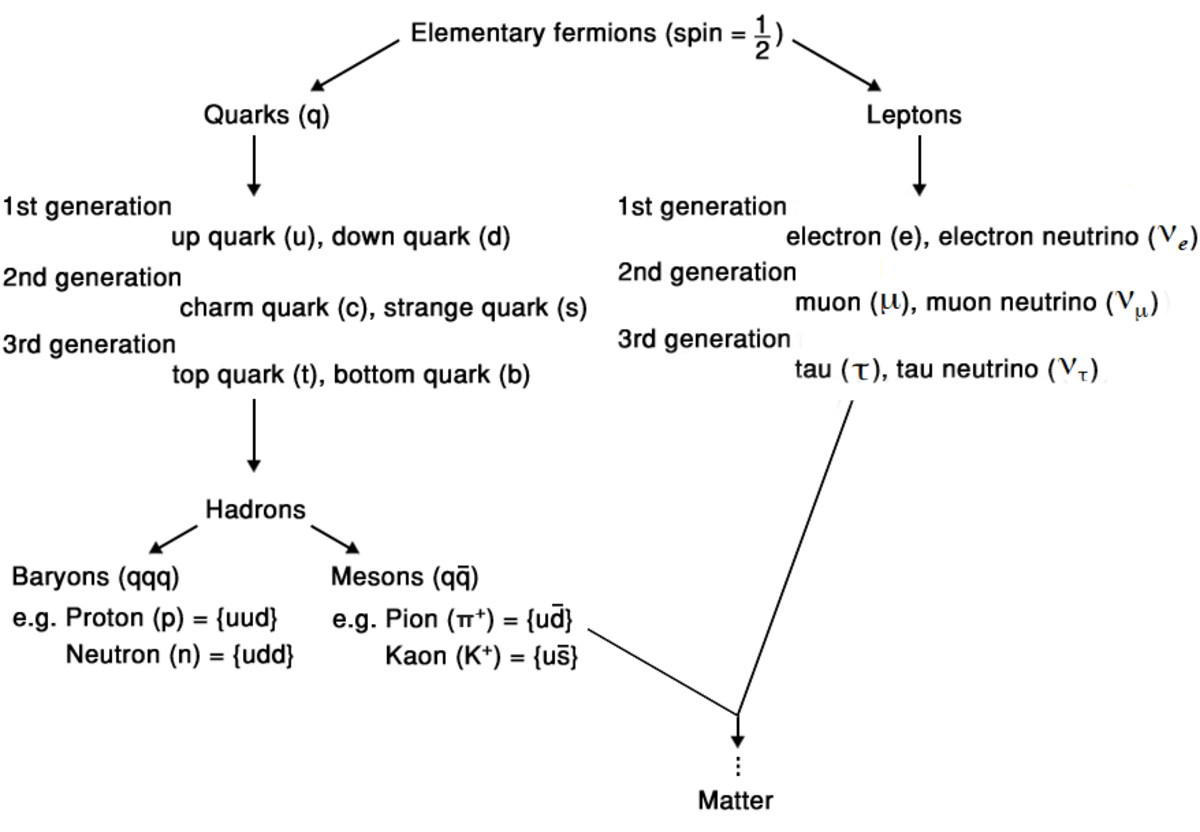The pattern of the twelve elementary fermions, how they are arranged into generations and then bind together to form matter, such as atoms.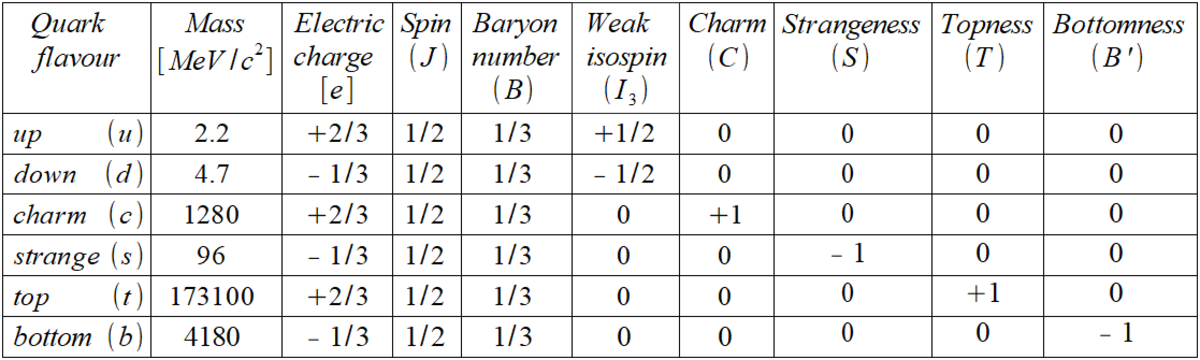A table showing the properties of the different flavours of quark. Mass is given in energy style units and electrical charge is given in units of the magnitude of the electron's charge.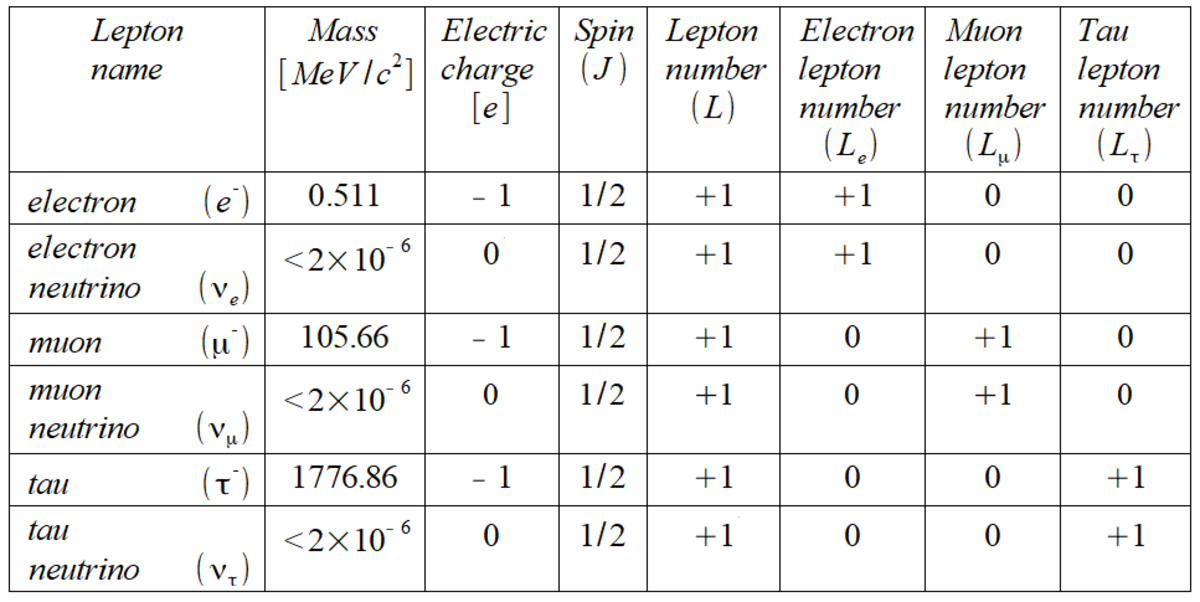A table showing the properties of the different types of lepton. Again, mass is given in energy style units and electrical charge is given in units of the magnitude of the electron's charge.

## Antimatter

Every matter particle has a partner, called its antiparticle. Antiparticles have almost all their properties the same as their partner, such as mass and lifetime. However, their charges are the opposite sign to their partner's charges. For example, the up antiquark has the same mass and spin as an up quark but its electric charge is -2/3 e, its baryon number is -1/3 and its weak isospin is -1/2. An antiparticle is symbolically denoted by a bar above the symbol for it's regular partner.

When a particle collides with its corresponding antiparticle they annihilate each other, the two particles are destroyed and converted into pure energy. This energy will then go on to create new particle-antiparticle pairs. Apart from this strange behaviour, antiparticles behave pretty normally, in the sense that they seem to interact with gravity in the same way as standard particles and antiparticles can bind together to form antimatter such as antibaryons, antiatoms and so on.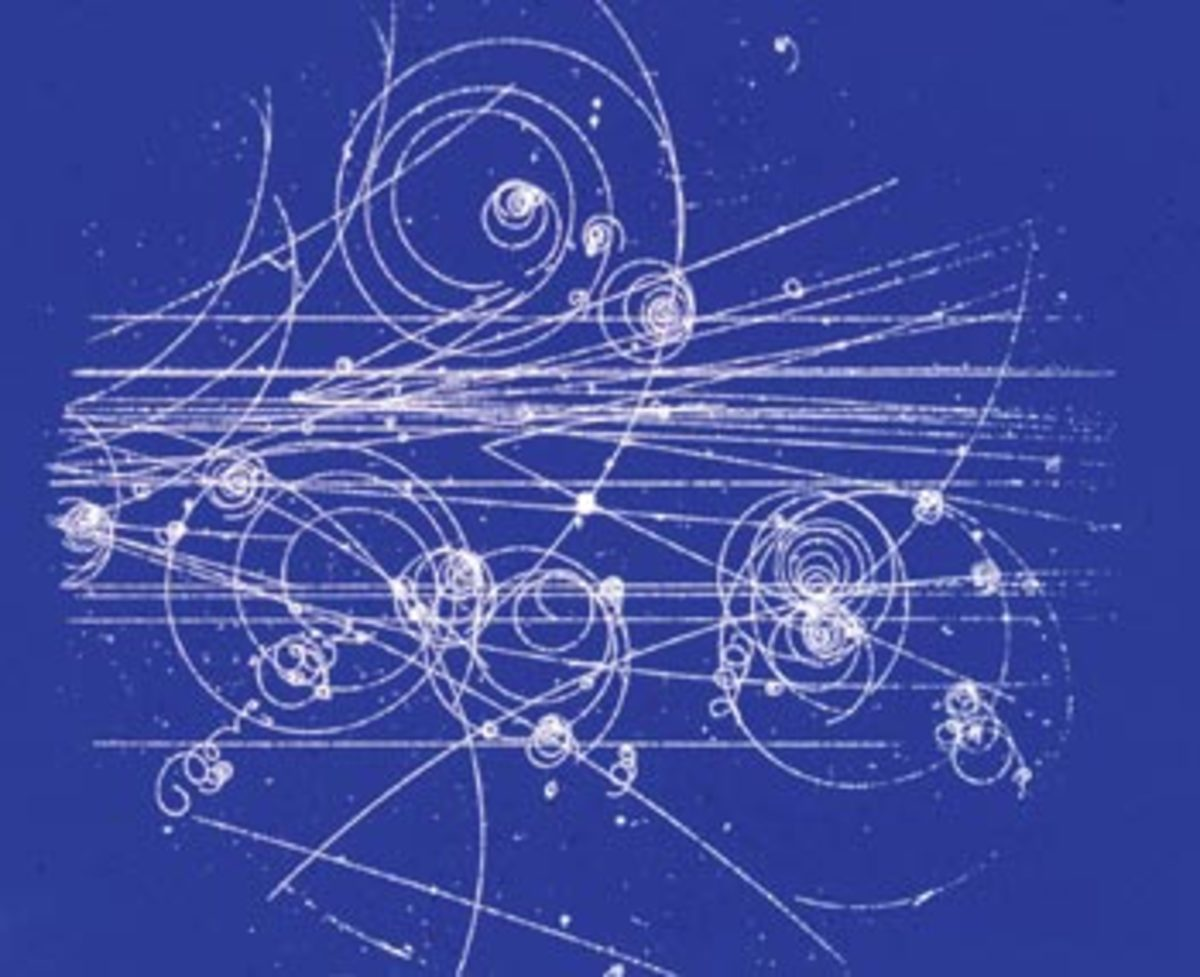An example of tracks left by particles in a bubble chamber. The first antiparticle discovered, the positron, was discovered by observing similar tracks. The positron track was identical to an electron's but spiralling in the opposite direction.CERN Courier

## Exchange Forces

The fundamental interactions (also interchangeably called "forces") of nature are caused by elementary fermions exchanging force carrier particles. Precisely, the exchange of virtual bosons. But what is a virtual particle? The underlying mathematical framework describing interactions between particles is quantum field theory (QFT). In QFT, particles are well defined excitations of quantum fields. Virtual particles are fluctuations in a field, that only exists for a limited time. These fluctuations are allowed, through quantum mechanics, to break energy conservation but this limits the time that the particle is allowed to exist, as described by the uncertainty principle.Heisenberg's uncertainty principle, a cornerstone of quantum mechanics, that relates the uncertainty in energy with the uncertainty in time.

Combining the uncertainty principle with Einstein's principle of mass-energy equivalence can then provide estimates for the lifetime of a virtual particle and its maximum possible range of motion in that time. If this virtual particle is a force carrier, these provide rough estimates of the range of an interaction and the characteristic time for it to take effect. For example, an interaction caused by a heavy exchange particle will have a shorter range than a light exchange particle.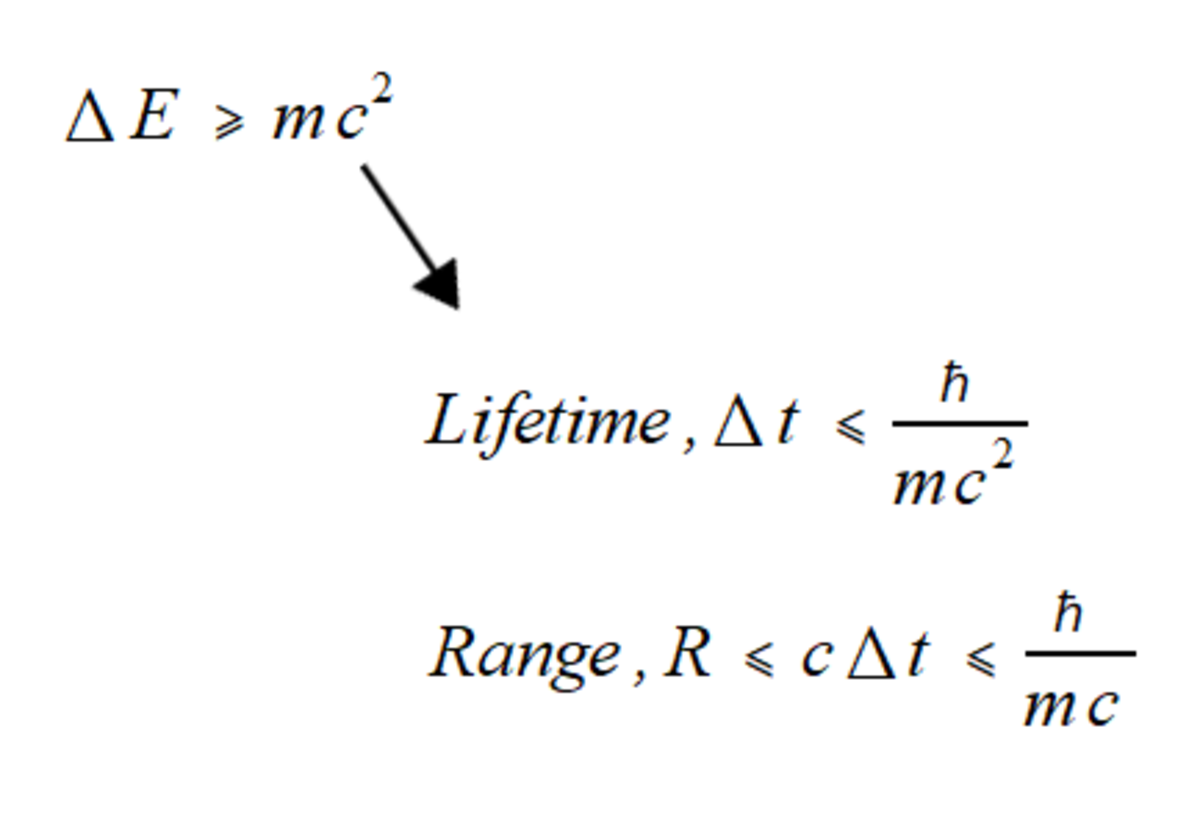The maximum range is estimated by assuming the force carrier moves at the speed of light.

There are only four fundamental interactions that have been observed within our universe: gravity, electromagnetism, the strong interaction and the weak interaction.

## Gravity

Gravity is the only fundamental interaction unexplained within the standard model. Our current model of gravity is Einstein's theory of general relativity. However, general relativity hasn't been reconciled with QFT. This isn't a large practical problem because gravity is the weakest force by a significant margin and gravitational forces can be assumed as negligible for interactions between particles.

## Electromagnetism

The electromagnetic interaction affects all particles that carry an electrical charge. It causes like charged particles to repel each other and oppositely charged particles to attract each other. The force carrier for electromagnetism is the photon (γ). The photon is electrically neutral and massless, hence the electromagnetic interaction has an infinite range (although it does get significantly weaker with distance). The characteristic time for electromagnetic interactions is somewhere between 10-20 and 10-15 seconds.

Examples of the electromagnetic interaction include atoms being held together, the force preventing stuff from falling through the floor, friction between objects and the interactions with electrons that form the basis of all electrical technology. All everyday phenomena are either produced by electromagnetic or gravitational interactions.

## Strong Interaction

Analogous to how the electromagnetic interaction couples to electrical charge, the strong interaction couples to a different kind of charge, the colour charge. All quarks carry a colour, which is either red, blue or green. Antiquarks can carry anti-red, anti-blue or anti-green. This has nothing to do with the quark's visible colour, it is simply another quantum number. All observable particles (ie. hadrons) have to be colour neutral. Mesons can obviously meet this requirement e.g. red + anti-red is colour neutral. Baryons can also be colour neutral if each quark is a different colour (an RGB combination), this is a labelling concept that draws from white light being a sum of different coloured lights.

The force carrier for strong interactions is the gluon (g). Gluons are massless and they also carry colour charges, meaning gluons can strongly interact with each other, unlike photons. A gluon carries both a colour and an anti-colour, this leads to there being eight different gluon types. The strong interaction is attractive but it gets stronger with distance. This effect leads to the phenomena of colour confinement. When attempting to pull apart quarks, the increasing distance between them increases the energy of the colour field between them. Eventually, it becomes a high enough energy that a quark-antiquark pair is created. This phenomenon prevents individual quarks from ever being observed.

Even though gluons are massless, the effect of colour confinement limits the range of the strong interaction to about 10-15 metres, roughly the size of the nucleus within an atom. The characteristic time for strong interactions is less than 10-20 seconds. At the atomic scale, the strong interaction is the dominant interaction and hence it is responsible for binding together quarks into hadrons and holding the nucleus together.

## Weak Interaction

The weak interaction causes massive particles to decay into lighter particles. Unlike the electromagnetic and strong interactions, the weak interaction is allowed to break the conservation of some quantum numbers such as strangeness. Breaking these conservation laws allows the weak interaction to change the flavour of quarks and so it can cause quarks to decay between generations. However, electrical charge, baryon number and lepton numbers must still be conserved.

The weak interaction has three force carriers: the W+, the W- and the Z0. All three particles have heavy masses (around 80 GeV/c2) which limits the range of the interaction to about 10-18 metres, significantly smaller than the size of a proton. The characteristic time for weak interactions is typically longer than 10-15 seconds. The weak nuclear interaction is responsible for nuclear decays and hence it is the fundamental interaction behind radioactivity.

Within the standard model, the weak interaction has been successfully unified with the electromagnetic interaction. Essentially, electromagnetism and the weak interaction are actually manifestations of the same interaction, the electroweak interaction. At our universe's current low energy they appear to be very different but at a high enough energy (around 246 GeV) they merge to become the same interaction.

## Higgs Boson

In the 1960s, Peter Higgs predicted a field permeating the universe to explain where the mass of standard model particles came from. Interactions with the Higgs field gives mass to all of the elementary particles in the standard model and as such is hugely important for the standard model to work. The higgs boson (H) is an excitation of the predicted Higgs field. Searching for the boson was the easiest way of confirming the Higgs field theory and hence a search for the Higgs boson was a long term ambition for particle physics. In 2012, a particle matching the properties expected of the standard model Higgs boson was discovered at the LHC. This was the final discovery of a particle in the standard model and a confirmation of the model.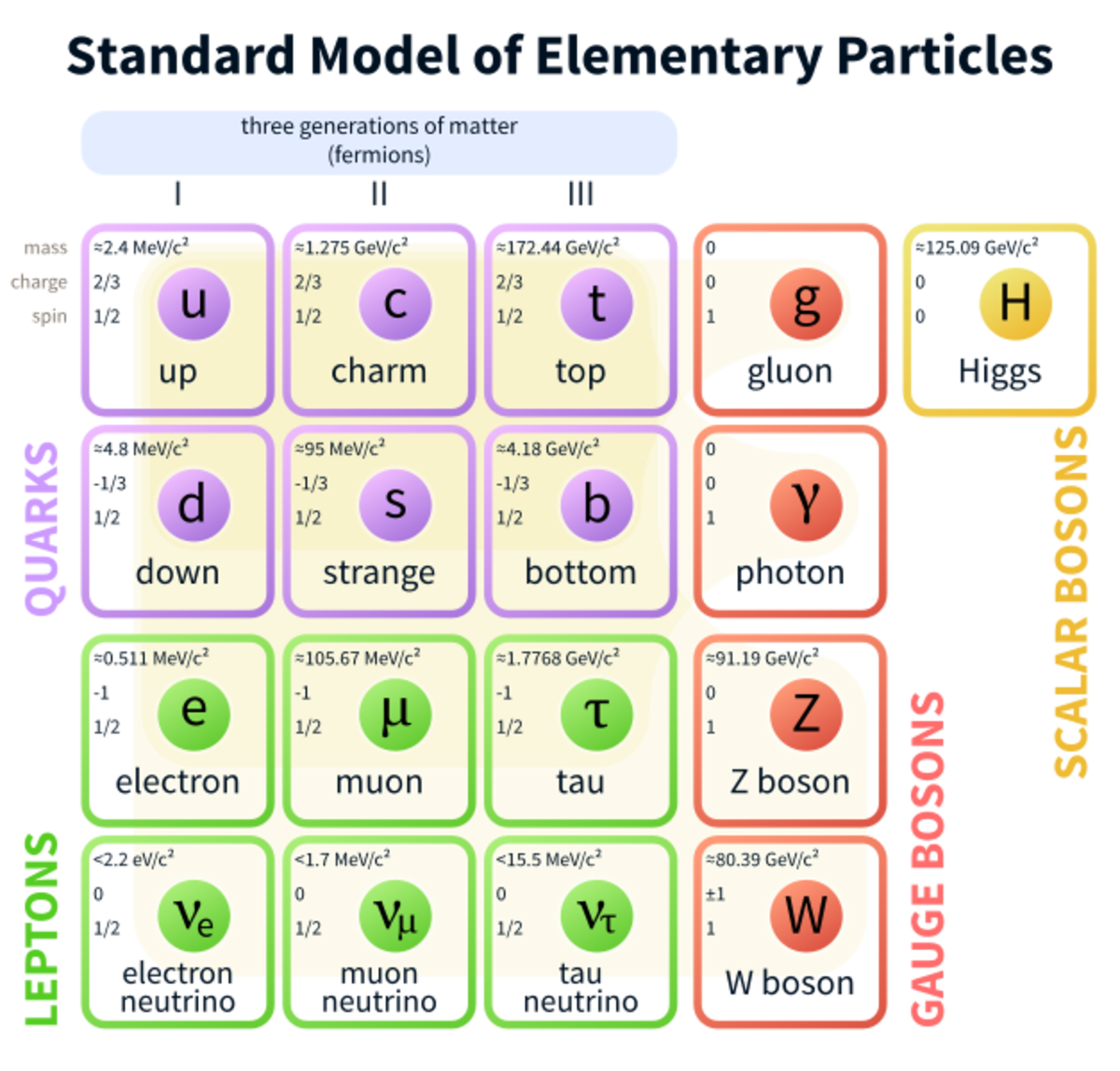A table showing all of the elementary particles in the standard model. Remember that all the leptons and quarks have a corresponding antiparticle.Wikimedia commons

## Feynman Diagrams

Feynman diagrams are simple, visual representations of particle interactions. They are used by theoretical particle physicists to calculate the probability of a specific interaction between a set of initial particles that leads to a set of final particles. A feynman diagram only shows one possible arrangement of particles that can produce the interaction. To calculate the total probability of the interaction occurring, a sum must be performed over all the possible arrangements that lead to the interaction. The use of feynman diagrams make these extremely complicated calculations more tangible and easier to digest.

Within the diagram, the trajectory of particles are represented by lines (either straight, dotted or squiggly depending on the type of particle). Vertices are where the particles meet and interact. Antiparticles are represented as travelling backwards in time. Virtual particles are represented by internal lines, lines which connect two vertices (starting and ending within the diagram). Real particles are represented by lines that either point into the diagram or out of the diagram.

Below are some examples of Feynman diagrams for a variety of particle interactions.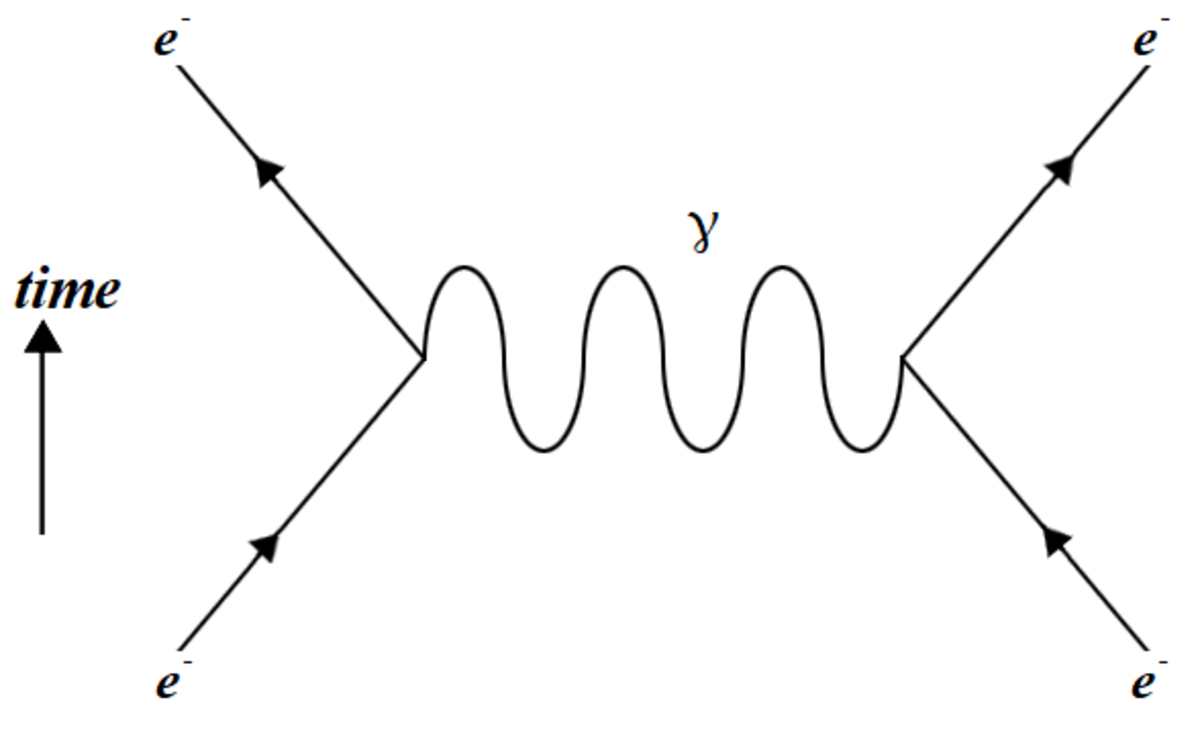Electromagnetic repulsion between two electrons. Notice the exchange of a virtual photon that gives rise to the repulsion.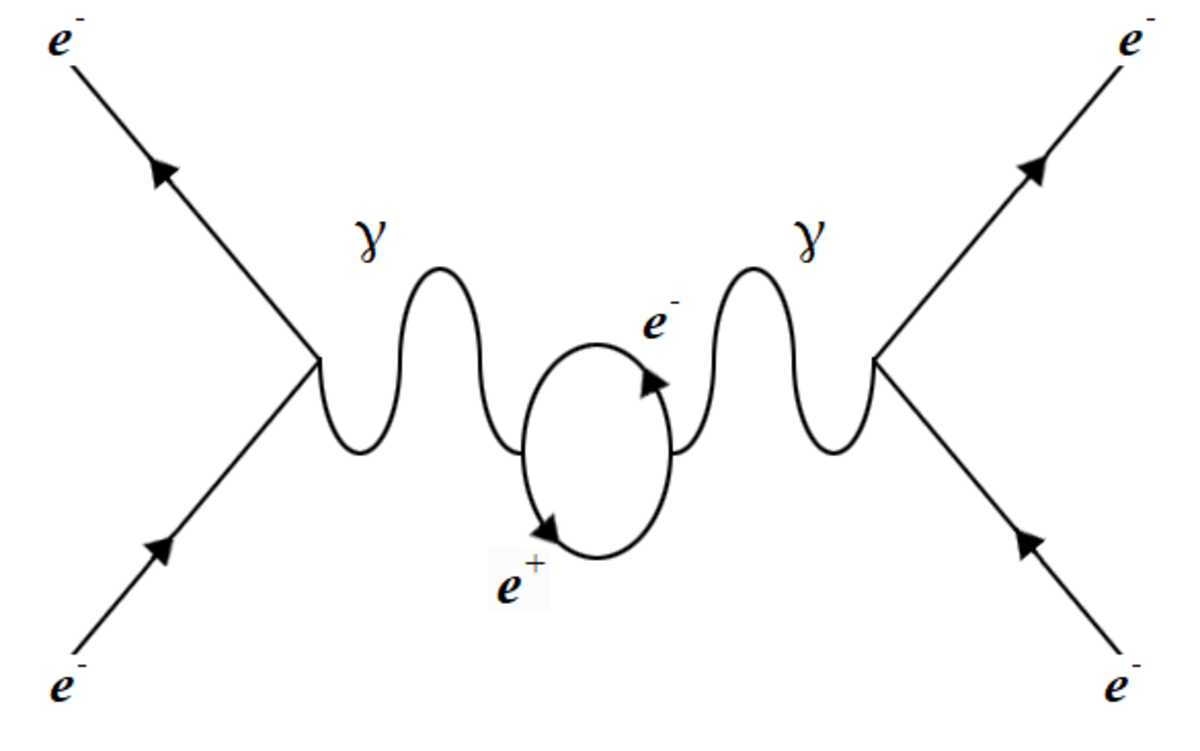A less probable arrangement that produces the same repulsion as above. In this case the photon creates a virtual electron-positron pair while travelling between the electrons.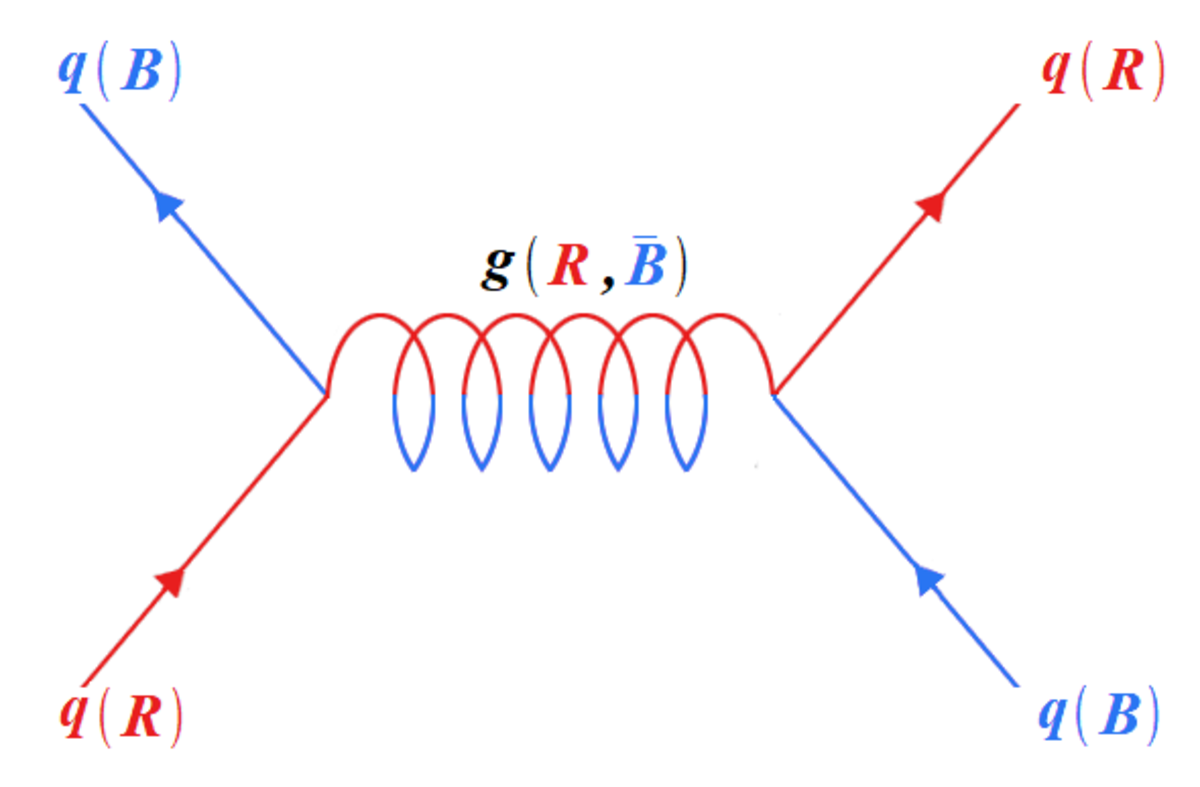The exchange of a gluon between two quarks, creating a strong force between them. Notice the conservation of overall colour. Colour is displayed in brackets next to each particle.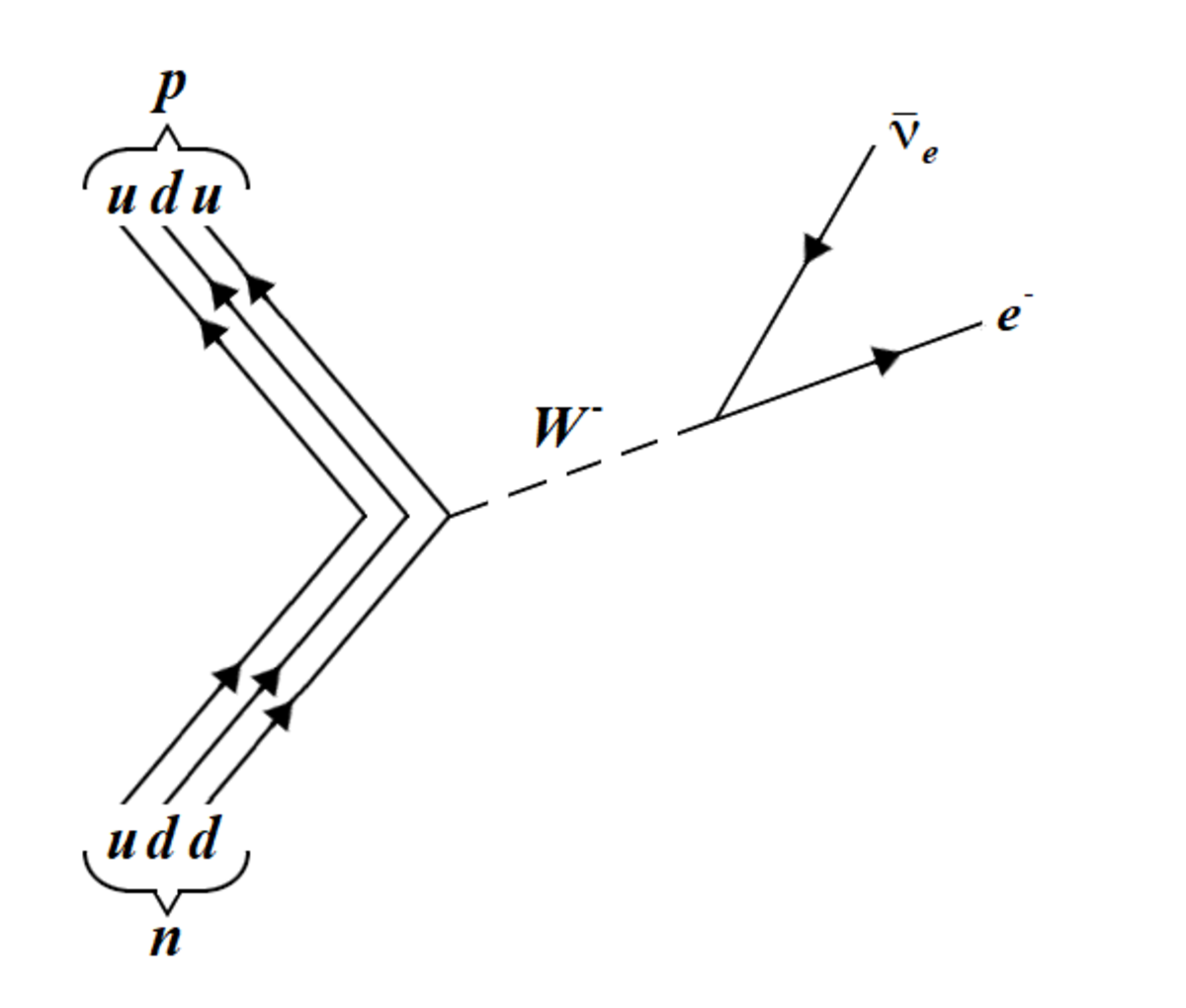The beta decay of a neutron into a proton, through the weak interaction.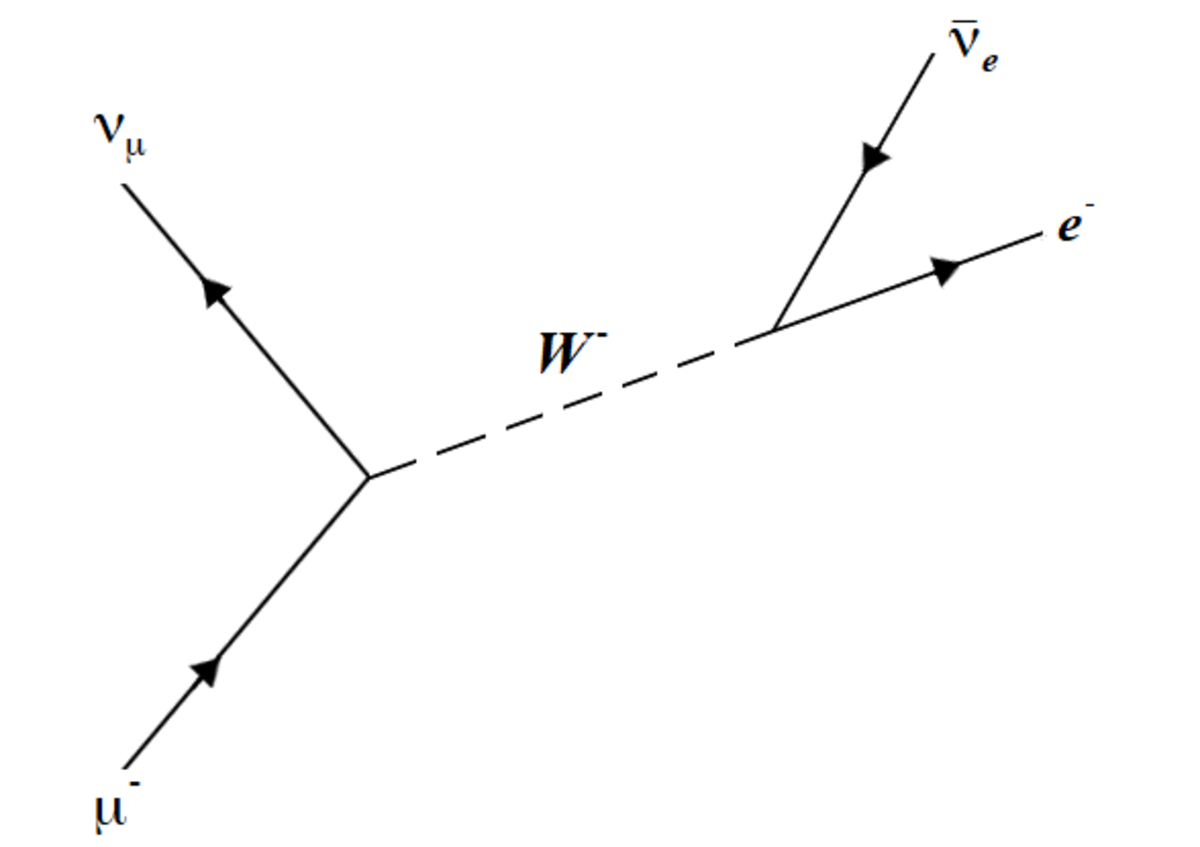The decay of a muon, also through the weak interaction. Notice the conservation of total lepton number as well as the individual electron and muon lepton numbers.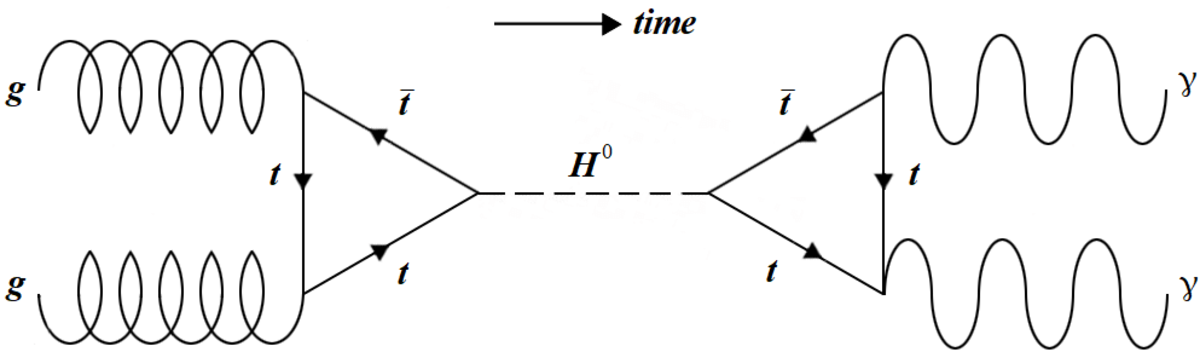The production of a Higgs boson via gluon fusion followed by a subsequent decay into two photons. These were some of the processes observed by search at the LHC. The time axis has switched to an horizontal orientation for convenience.

## Limitations of the Standard Model

Despite being a successful, thoroughly tested theory we know that there are problems the theory can't explain. These gaps in our understanding indicate that the standard model is only an approximation (at low energies) of a more complete theory.

These are currently the major unsolved problems within the standard model:

• Neutrino mass - The standard model initially assumed neutrinos were massless, until it was experimentally determined that they had some tiny mass. Their exact masses and the mechanism behind is not yet understood.
• Interaction unification - Two of the four fundamental interactions have already been unified into the electroweak interaction. The next step is to try and unify this with the strong force, at an even higher unification energy. The aim would then be to unify this with gravity and have one fundamental unified interaction.
• Gravity - Gravity isn't explained within the standard model. The properties of a graviton, the name coined for a potential force carrier, are still debated let alone observed by experiment. Most likely, a new model is needed to reconcile quantum mechanics with gravity; one of many potential candidates is string theory.
• Dark matter and dark energy - Only about 5% of our universe consists of 'ordinary matter' as explained by the standard model. The rest is made up of completely unexplained kinds of matter and energy, which we currently call dark matter and dark energy.
• Matter-antimatter asymmetry - We observe that our universe is almost exclusively matter, where is all the antimatter? The standard model gives no reasons why antimatter and matter shouldn't be produced in equal amounts. Therefore, it is a question of cosmology and particle physics as to why our universe has developed into such an asymmetrical state.